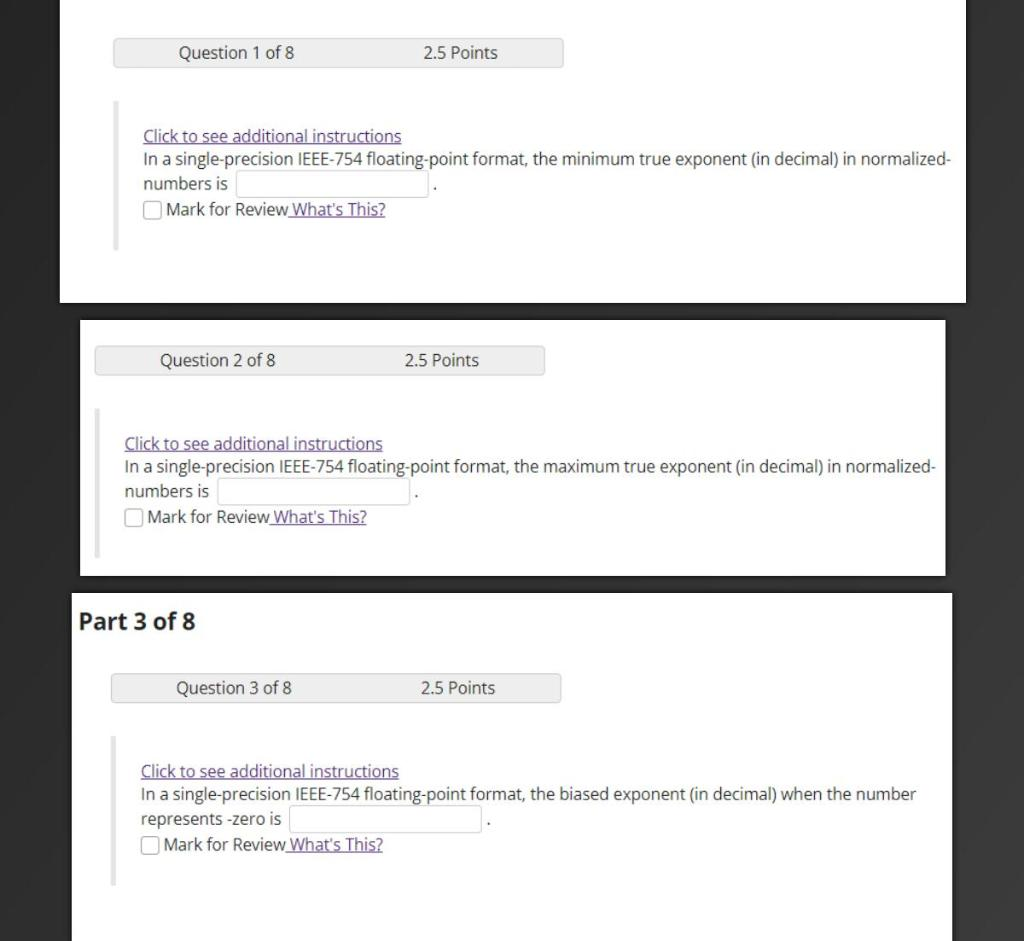# Question Solved1 AnswerQuestion 1 of 8 2.5 Points Click to see additional instructions In a single-precision IEEE-754 floating point format, the minimum true exponent (in decimal) in normalized- numbers is Mark for Review What's This? Question 2 of 8 2.5 Points Click to see additional instructions In a single-precision IEEE-754 floating-point format, the maximum true exponent (in decimal) in normalized- numbers is Mark for Review What's This? Part 3 of 8 Question 3 of 8 2.5 Points Click to see additional instructions In a single-precision IEEE-754 floating point format, the biased exponent (in decimal) when the number represents -zero is Mark for Review What's This?CKSVGG The Asker · Computer ScienceTranscribed Image Text: Question 1 of 8 2.5 Points Click to see additional instructions In a single-precision IEEE-754 floating point format, the minimum true exponent (in decimal) in normalized- numbers is Mark for Review What's This? Question 2 of 8 2.5 Points Click to see additional instructions In a single-precision IEEE-754 floating-point format, the maximum true exponent (in decimal) in normalized- numbers is Mark for Review What's This? Part 3 of 8 Question 3 of 8 2.5 Points Click to see additional instructions In a single-precision IEEE-754 floating point format, the biased exponent (in decimal) when the number represents -zero is Mark for Review What's This?
More
Transcribed Image Text: Question 1 of 8 2.5 Points Click to see additional instructions In a single-precision IEEE-754 floating point format, the minimum true exponent (in decimal) in normalized- numbers is Mark for Review What's This? Question 2 of 8 2.5 Points Click to see additional instructions In a single-precision IEEE-754 floating-point format, the maximum true exponent (in decimal) in normalized- numbers is Mark for Review What's This? Part 3 of 8 Question 3 of 8 2.5 Points Click to see additional instructions In a single-precision IEEE-754 floating point format, the biased exponent (in decimal) when the number represents -zero is Mark for Review What's This?